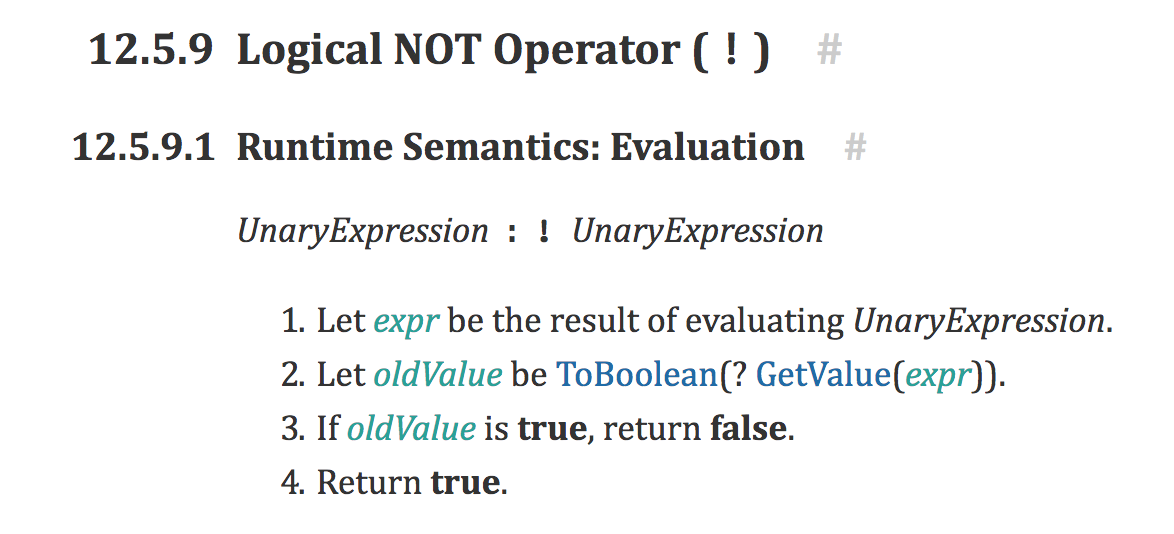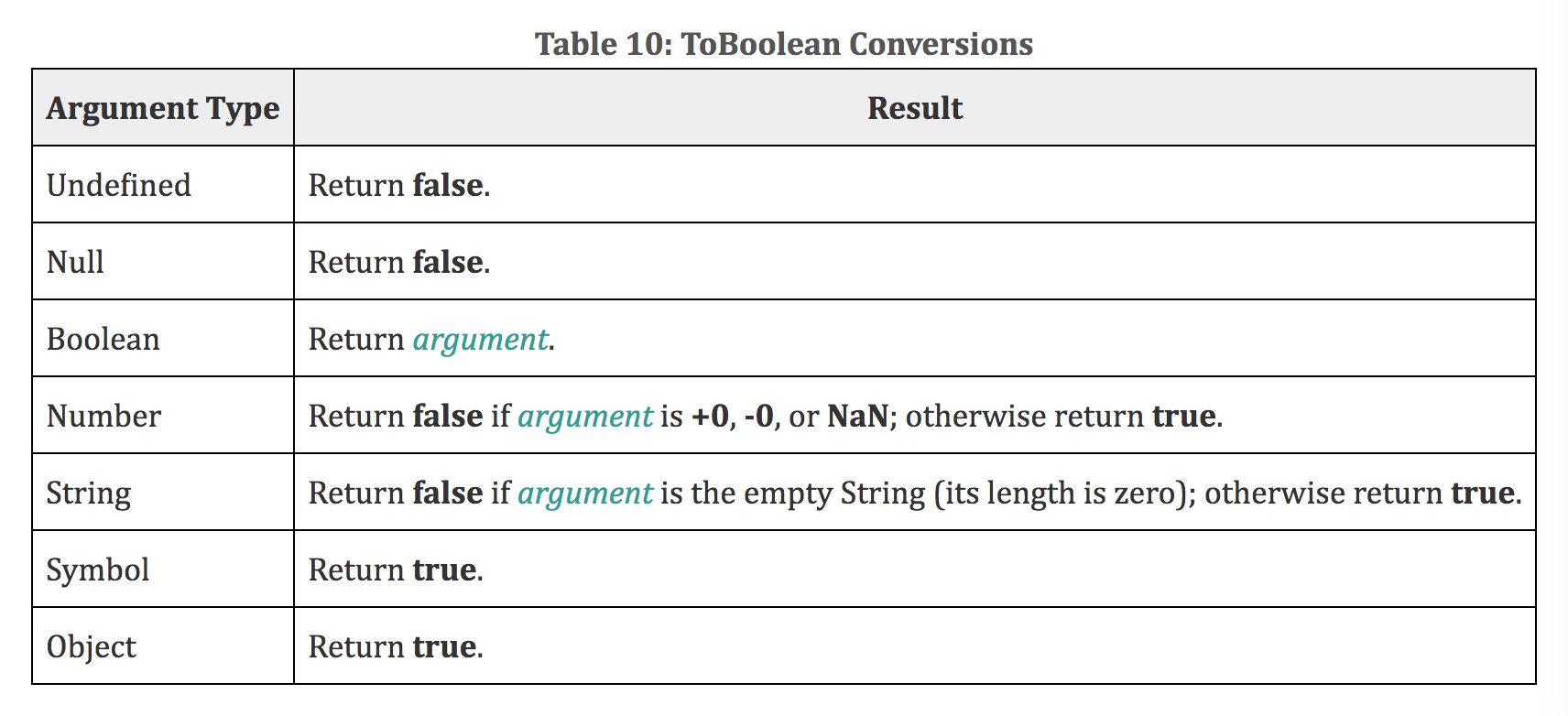“征服 JavaScript 面试”是我写的一系列文章，来帮助面试者准备他们在面试 JavaScript 中、高级职位中将可能会遇到的一些问题。这些问题我自己在面试中也经常会问。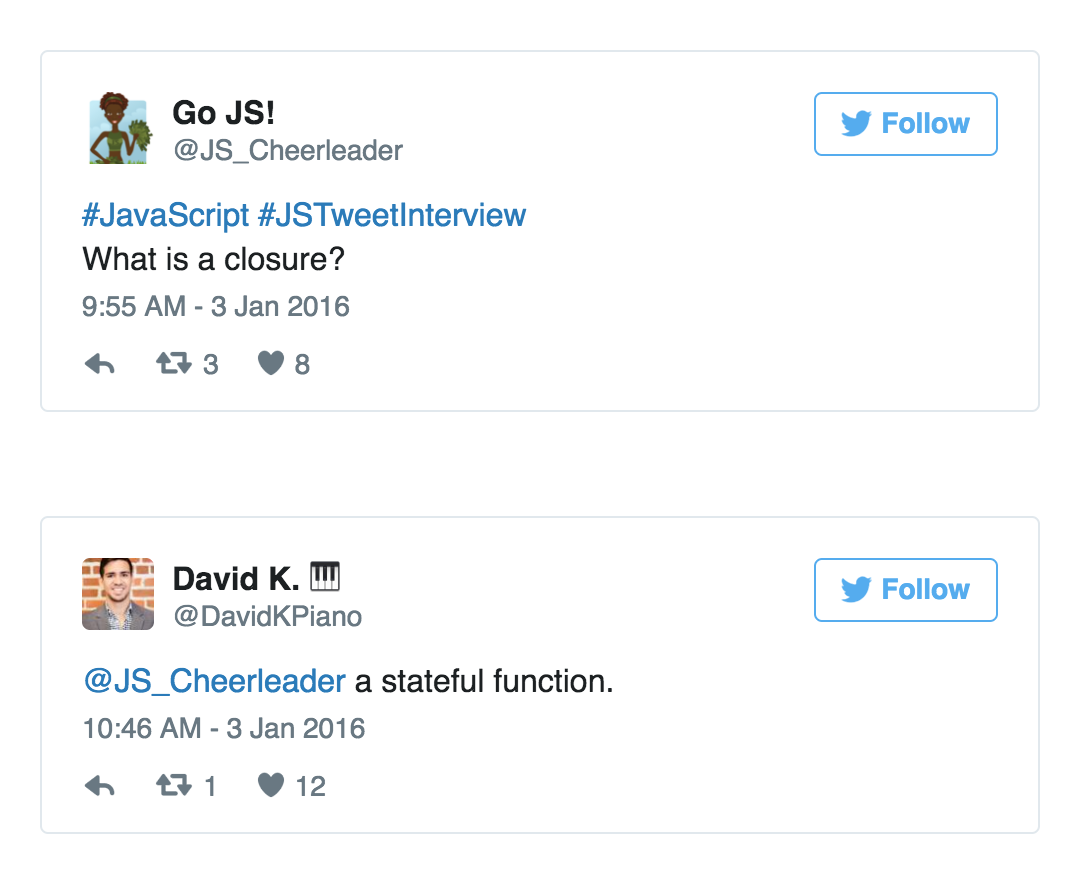### 闭包使用的例子

“面向接口编程，别面向实现编程。” 设计模式：可复用面向对象软件的要素

const getSecret = (secret) => {
return {
get: () => secret
};
};

test("Closure for object privacy.", assert => {
const expected = 1;
const obj = getSecret(1);

const actual = obj.get();

try {
assert.ok(secret, "This throws an error.");
} catch (e) {
assert.ok(true, The secret var is only available
to privileged methods.);
}

assert.equal(actual, expected, msg);
assert.end();
});


const secret = (msg) => () => msg;

// Secret - creates closures with secret messages.
// https://gist.github.com/ericelliott/f6a87bc41de31562d0f9
// https://jsbin.com/hitusu/edit?html,js,output

// secret(msg: String) => getSecret() => msg: String
const secret = (msg) => () => msg;

test("secret", assert => {
const msg = "secret() should return a function that returns the passed secret.";

const theSecret = "Closures are easy.";
const mySecret = secret(theSecret);

const actual = mySecret();
const expected = theSecret;

assert.equal(actual, expected, msg);
assert.end();
});


partialApply(targetFunction: Function, ...fixedArgs: Any[]) =>
functionWithFewerParams(...remainingArgs: Any[])


partialApply 接受一个多参数的函数，以及一串我们想要提前赋给这个函数的参数，它返回一个新的函数，这个函数将接受剩余的参数。

const add = (a, b) => a + b;


const add10 = partialApply(add, 10);


// Generic Partial Application Function
// https://jsbin.com/biyupu/edit?html,js,output
// https://gist.github.com/ericelliott/f0a8fd662111ea2f569e

// partialApply(targetFunction: Function, ...fixedArgs: Any[]) =>
//   functionWithFewerParams(...remainingArgs: Any[])
const partialApply = (fn, ...fixedArgs) => {
return function (...remainingArgs) {
return fn.apply(this, fixedArgs.concat(remainingArgs));
};
};

const msg = "partialApply() should partially apply functions"

const add = (a, b) => a + b;

const expected = 15;

assert.equal(actual, expected, msg);
});


### 轮到你来试试了

console.log([] == false, !![] == true); // true, true来源：http://www.ecma-international.org

• 第 7 条：If Type(y) is Boolean, return the result of the comparison x == ToNumber(y).
• 第 9 条：If Type(x) is Object and Type(y) is either String, Number, or Symbol, return the result of the comparison ToPrimitive(x) == y.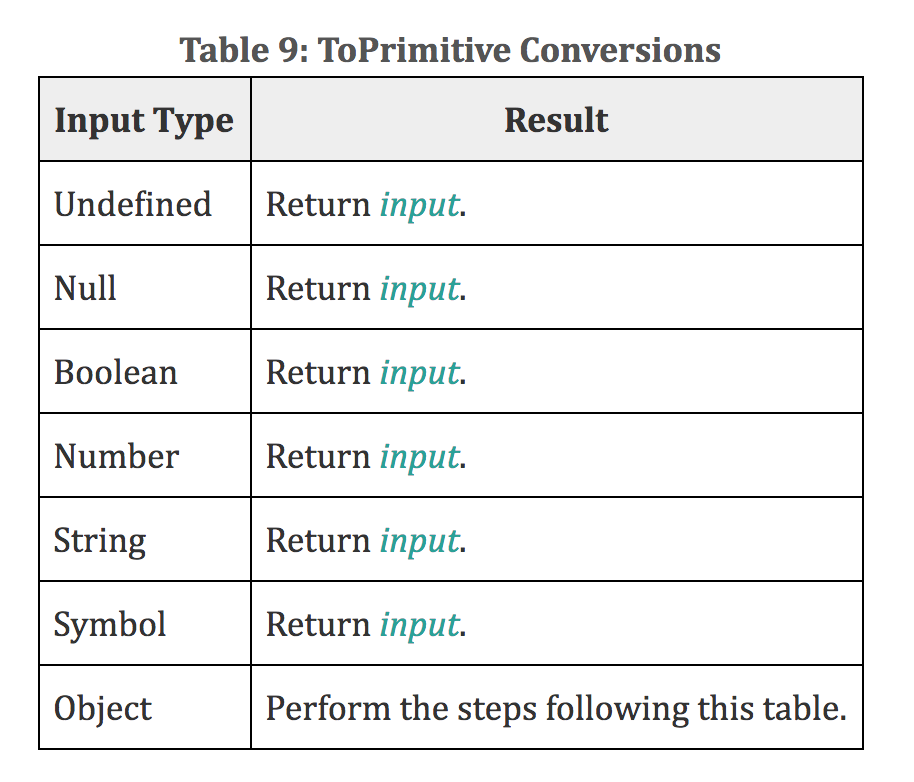来源：http://www.ecma-international.org来源：http://www.ecma-international.org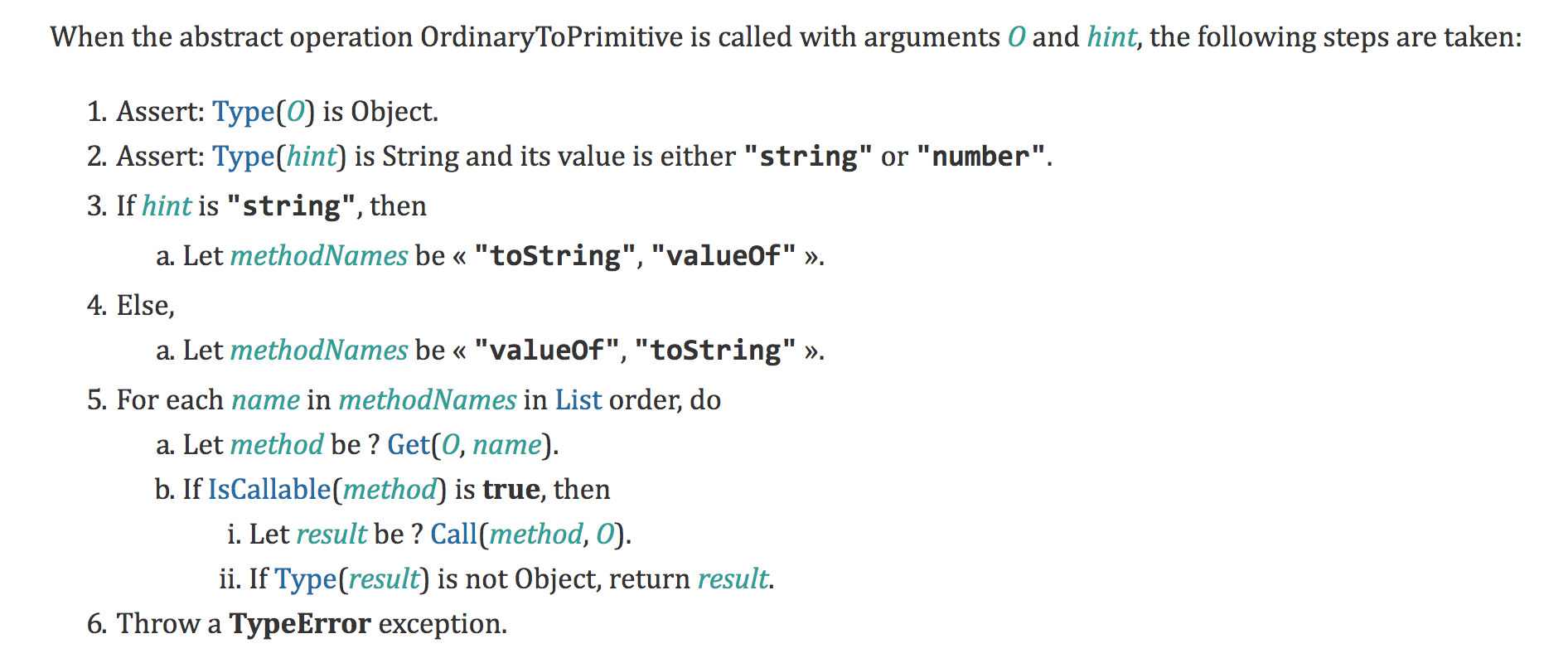来源：http://www.ecma-international.org